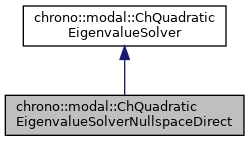## Description

Solves the eigenvalue problem with a direct method: first does LU factorization of Cq jacobians to find the null space, then solves the problem using the direct Eigen::EigenSolver.

Note: since intermediate dense matrices are built, the performance is acceptable only for small-sized problems. Note: since the method is direct, all eigenvalues are computed, regardless of n_modes, but only lower n_modes are returned.

#include <ChEigenvalueSolver.h>[legend][legend]

## Public Member Functions

virtual bool Solve (const ChSparseMatrix &M, const ChSparseMatrix &R, const ChSparseMatrix &K, const ChSparseMatrix &Cq, ChMatrixDynamic< std::complex< double >> &V, ChVectorDynamic< std::complex< double >> &eig, ChVectorDynamic< double > &freq, ChVectorDynamic< double > &damping_ratio, ChEigenvalueSolverSettings settings=0) const override
Solve the quadratic eigenvalue problem (lambda^2*M + lambda*R + K)*x = 0 s.t. More...

## ◆ Solve()

 bool chrono::modal::ChQuadraticEigenvalueSolverNullspaceDirect::Solve ( const ChSparseMatrix & M, const ChSparseMatrix & R, const ChSparseMatrix & K, const ChSparseMatrix & Cq, ChMatrixDynamic< std::complex< double >> & V, ChVectorDynamic< std::complex< double >> & eig, ChVectorDynamic< double > & freq, ChVectorDynamic< double > & damping_ratio, ChEigenvalueSolverSettings settings = 0 ) const
overridevirtual

Solve the quadratic eigenvalue problem (lambda^2*M + lambda*R + K)*x = 0 s.t.

Cq*x = 0 If n_modes=0, return all eigenvalues, otherwise only the first lower n_modes.

Parameters
 M input M matrix R input R matrix K input K matrix Cq input Cq matrix of constraint jacobians V output matrix with eigenvectors as columns, will be resized eig output vector with complex eigenvalues (real part not zero if some damping), will be resized freq output vector with n frequencies [Hz], as f=w/(2*PI), will be resized. damping_ratio output vector with n damping rations r=damping/critical_damping. settings optional: settings for the solver, or n. of desired lower eigenvalues. If =0, return all eigenvalues.